Since 2013

• 100% Quantified, data-driven and Backtested
• We always show our results!
• Signals every day via our site or email
• Cancel at any time!

# Linear vs. Logarithmic Chart Scaling Explained …What is the difference, and which is better?

Last Updated on 11 September, 2023 by Samuelsson

The price movements of an asset can be viewed in different scales — linear or logarithmic scale — and most modern trading platforms can display in any scale. Determining the best chart scaling method to display the price action can help you improve your analysis and trading results. But what is the difference between the linear and logarithmic scales, and which is better?

In the logarithmic chart scale, the prices (Y-axis) are spaced proportionally and not equidistantly, while in the linear scale, the prices are spaced equidistantly — an equal value between price positions. The logarithmic scale is better for showing wide price differences over a long period, but you will prefer the linear scale when you’re dealing with small price fluctuations over a short duration.

To help understand the topic, we will discuss it under the following headings:

• Linear Chart Scaling
• Logarithm Chart Scaling
• Benefits of logarithm chart scaling
• Differences between linear and logarithm chart scaling
• How changing the chart scaling can affect some technical tools
• Which chart scaling should you choose?
• Comparing logarithmic vs. linear scale for equity curves

## Linear Chart Scaling

In a price chart, the price is represented on the y-axis, while the time is represented on the x-axis. Our focus is on how the price axis is scaled. Here, we’ll discuss when to use linear chart scaling and why short-term traders prefer it. But first, let’s find out what linear scaling is.

### What is linear chart scaling?

Linear chart scaling uses an equal value between price positions on the y-axis. It plots price changes in a constant unit value such that any two price positions are equidistant from each other. The price spacing is equal, and the reference points along the y-axis ascend in equal increments. In other words, a unit precisely stands for a unit.

What this means is that a price change from \$1 to \$2 is represented just as the price change from \$100 to \$101. In the price change of \$1 to \$2, the stock doubled, but in moving from \$100 to \$101, the stock only gained one percent. This often creates challenges for securities that have multiplied in price. The situation of underrepresenting price changes that happen when security price is low (compared to the rest of the prices on the chart) and over-representing price changes when the security price is relatively high is the major problem associated with linear chart scaling.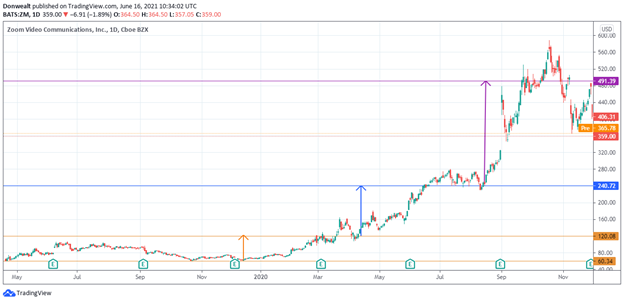Let’s take the linear chart of Zoom (ZM) above as an example. The price action in the portion of the chart with brown color seems static. But it represented the price doubling from \$60 to \$120. Each time, it takes a bigger price change for the price to double again, as can be seen in the blue and purple arrows. This is the main drawback of using a linear chart on either a long timeframe or once the security has doubled on the chart. In this case, for instance, because ZM was cheaper between \$40 and \$120 in the marked-portion, the price changes look insignificant.

### When to use linear chart scaling?

Linear chart scaling is used when a security price is moving within a narrow range or over a short time. In this situation, the trader wants to clearly see the small changes in price and know their actual value. Thus, the linear chart scale is best for short-term traders, such as scalpers, day traders, and swing traders, because these categories of traders are only concerned with price movements that happen over a short time — minutes, hours, days, or a few weeks — which are relatively small most of the time.

### Why do short-term traders prefer linear chart scaling?

Linear chart scaling has been criticized by many for being ineffective, but this is not the case at all. Linear chart scaling is preferred by short-term traders because it is nearly identical to semi-log scaling. In situations where the price range is constrained within a relatively narrow range, linear chart scaling can be very useful. Another reason why short-term traders prefer linear chart scaling is that price quotes for stocks are shown in absolute dollars, so the scale reflects price movements in dollars. Therefore, it makes sense for most short-term traders to use the linear scale in their chart so as to get the purest representation of price changes.

## Logarithm Chart Scaling

You may want to know what logarithmic chart scaling is, when to use it, and why long-term traders may prefer it. We will discuss all of that.

### What is logarithm chart scaling?

Instead of a unit of measure, logarithmic chart scaling shows the values proportions of the log. In other words, the price changes on the y-axis are not represented in their units but rather in a proportion — most commonly percentage — of the initial value. A logarithmic price scale is plotted such that the prices in the scale are not positioned equally from one another but rather represent an equal proportional increase (percentage increase) in the price of the security.

Since equal percentage price change is represented by the same distance on the price axis, it means that in a logarithmic chart of a stock, there will be the same distance on the y-axis when the stock goes from \$2 to \$4 and when it goes from \$80 to \$160. The percentage changes are denoted equally, the price notwithstanding. Thus, logarithmic chart scaling helps to capture the importance of the movement of larger prices. It is especially useful in analyzing exponential growth.

For instance, let’s take another look at Zoom (ZM). A linear chart scaling will not give the true picture of recent price changes since the stock crossed above the \$240, as you can see in the linear chat below; the price movement from \$240 to \$480 looks much more significant than the price movement from \$60 to \$240, although the \$240 to \$480 is only a 100% increase, relative to the \$60 to \$240 movement, which represents a 300% increase.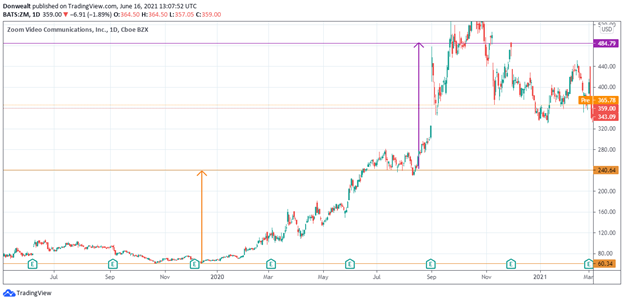If we compare this to the logarithmic chart below, you can see that the logarithmic chart puts the recent price movement into the right perspective, as it compares the entire price movement. The logarithmic scale gives a better and more accurate representation of the price movement in recent times. The more highly priced the stock is, the more suitable it is to use a logarithmic scale to ascertain the real impact of the price movement in terms of returns.### When to use logarithm chart scaling

It is better to use the logarithmic scale when the differences from the beginning and the end of the period are large. In this situation, the trader wants to put the recent price changes in perspective, irrespective of how apparently large it is. So, long-term traders who analyze the course of a price movement over months and years may want to use the logarithmic scale to put things in the right perspective.

By using both linear and logarithmic scales, long-term traders are able to see how the price fluctuates, both in dollar value and percentage yield. For example, the percentage change from \$50 to \$55 is the same as the percentage change from \$75 to \$82.50. In most cases, the scaling method used does not make a difference in less volatile stocks with short-to-intermediate-term time frames on their charts. It will not make much of a difference if you switch from linear to logarithmic chart scaling.

### Why do long-term traders prefer log chart scaling?

Long-term traders prefer logarithmic chart scaling because it allows them to see how the price has changed in percentage terms. It helps them to put things in the right perspective by studying the increment relative to the entire price movement. Since logarithmic scaling shortens the data points, it enables long-term traders who want to see the price movement over several months and years to get a broad view of the market.

## Benefits of logarithm chart scaling

Here are some of the benefits:

Presents a proportional representation of price movement and returns: In the linear chart scale, a move from \$100 to \$200 is represented by the same units (\$100) as a move from \$200 to \$300. However, in terms of percentage, the move from \$100 to \$200 represents a 100% return, while the move from \$200 to \$250 represents a 50% return. So, the logarithmic chart scale shows the price changes in terms of percentage returns.

Expresses a less severe move properly: Logarithmic chart scaling also tends to show less severe price increases or decreases than linear chart scaling. An example is if an asset has collapsed from \$100.00 to \$10.0. The distance between each dollar would be very small on a linear price scale, making it impossible to see a big move from \$15.00 to \$10.00. Logarithmic chart scaling solves these problems by adjusting the process based on the percent change. This means that, in logarithmic chart scaling, a significant percentage move will always correspond with a significant visual move.

Easily shows fast and significant price moves in the past: The first chart below uses a linear chart to represent Bitcoin (in USD). The movements before 2017 are not obvious in the chart. While the change appears dramatic from the logarithmic scale, the two charts display the same data. The rise in 2017 is extremely significant in the logarithm scale. Although this is not visible on the linear chart, it is noteworthy nonetheless. Using logarithmic chart scaling is thus always beneficial because of this. Using a logarithmic chart scaling method, you will be able to better cope with extreme skewness.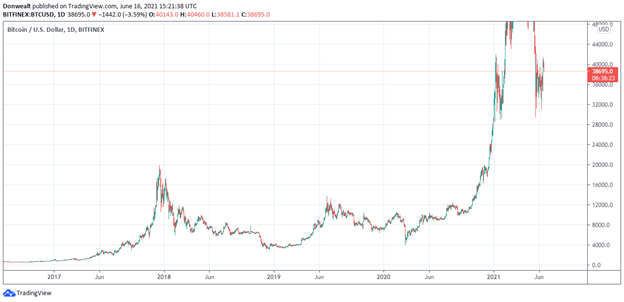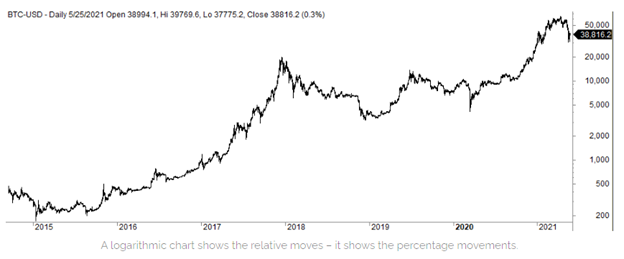## Differences between linear and logarithm chart scaling

A linear chart shows the absolute values changes, while a logarithmic scale shows the relative changes. To shed light on the key difference between logarithmic chart scaling and linear chart scaling, we will here use an example: On the linear chart, price increase from \$10 to \$15 is represented by the same upward movement in the same way as an increase between \$20 and \$25. The two increases are \$5 and the linear chart represents the price in equal segments.

For the logarithmic price scale, however, there is a different vertical movement for the changes in price between \$10 and \$15 as well as the change in price between \$20 and \$25. And although both are the same dollar amount move, the first \$5 change represents a 50% increase in the asset’s price. The second \$5 change represents a 25% increase in the asset’s price.

Because a 50% gain is more significant than 25%, a larger distance between the prices to clearly show the magnitude of the changes is often used by chartists. Using the logarithmic chart scaling, the vertical distance between the prices on the scale will be equal when the percent change between the values is the same. With this example, the distance between \$10 and \$15 would be equal to the difference between \$20 and \$30, yet they both represent a price increase of 50%. Herein is the key difference between logarithmic chart scaling and linear chart scaling.

Other differences between linear chart scaling and logarithmic chart scaling include:

• On a linear chart, each unit change is treated the same. The change from \$1 to \$2 looks the same from \$10 to \$11. On a logarithmic chart, on the other hand, it is the percentage change that is treated the same.
• Linear charts become useful when you want to see the pure price changes with scaling calculations. Day traders often prefer linear charts. Whereas logarithmic charts are useful when viewing long-term charts.
• Linear chart scaling givesabsolute values, while logarithmic chart scaling gives relative values.
• The linear price chart could have an equal distance of 5 units on the y-axis. The logarithmic chart displays the values using price scaling rather than a unique unit of measure.
• If a security has small price moves and choppy trading action, a linear chart would be the best method for charting the stock. Logarithm chart scaling is best for volatility in price moves and trading action.

## Which chart scaling should you choose?

Firstly, the price of a security is usually analyzed in relative terms. So, it may be best to represent the security’s price movement in percentage terms rather than in absolute terms. This is one of the reasons logarithmic chart scaling is recommended for analyzing returns. Logarithmic chart scaling gives relative values, while linear chart scaling gives absolute values.

When the price of the stock you want to track has moved significantly over the time your chart covers, a logarithmic scale is invaluable. For example, if the stock’s price has dropped from \$150 to \$8, and you use an arithmetic scale, you’ll have to create extremely close dollar increments, as you’ll have to view your graph on a large screen. With this in mind, you won’t notice the 12.5% change from \$8 to \$9. This problem will be avoided using a logarithmic scale. A significant percentage of movement is linked to a significant visual change regardless of where you are on the chart.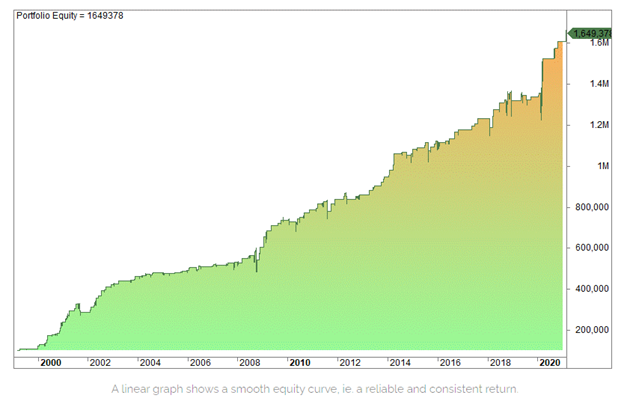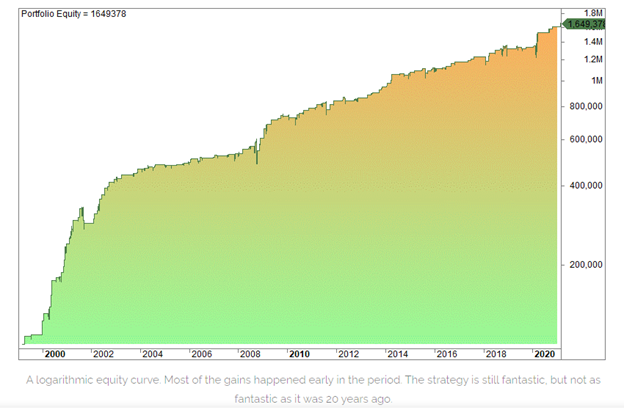## Comparing logarithmic vs. linear scale for equity curves

Let’s say that you are conducting a test over a decade, such as between 1990 and 2021, and you can expect to earn 12% CAGR during this period. However, the performance could be entirely different from the beginning and end date. It might have been an excellent strategy in the 1990s, but it has subsequently gone awry in the preceding five to six years, for instance, from 2015. If a linear equity curve is used, the disparity between the points won and lost will be invisible and may even mislead one into thinking the strategy is still working like before.

To demonstrate this, let’s see the example below of how equity curves can differ depending on whether a strategy employs a linear or logarithmic scale. The strategy is tested on Nasdaq/QQQ and has an average gain of 3.21% per trade from 1999 till the later end of 2005. The average gain is just 1.16% between 2006 and 2021. Although this strategy is still doing fantastic, it was more successful at the beginning during the dot-com era of high volatility, despite being a long-only strategy.

See how the strategy’s performance looks on a linear chart:

Now, see how the performance looks on a logarithmic scale:

As you can see, the logarithmic scale shows that the strategy’s performance has dropped by a lot.

## Final words

A logarithmic chart scaling uses the percentage of change to plot data points. The data points on the y-axis in logarithmic chart scaling are not positioned equidistantly. A linear chart scale, on the other hand, uses an equal value between price points.

While logarithmic chart scaling gives relative values, linear chart scaling gives absolute values. Short-term traders prefer linear chart scaling because the price is often constrained within a relatively narrow range over the short term. Logarithmic chart scaling is more useful to long-term traders because it shows percentage price returns and can compress enormous price data into one chart view.